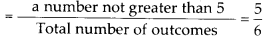## MP Board Class 8th Maths Solutions Chapter 5 Data Handling Ex 5.3

Question 1.
List the outcomes you can see in these experiments.
(a) Spinning a wheel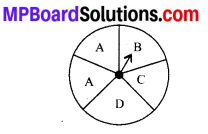(b) Tossing two coins together.
Solution:
(a) After spinning a wheel, we obtained total outcomes: A, B, C and D.
(b) After tossing two coins together, we get the following outcomes:
(i) HT i.e., Head on first coin and Tail on the second coin.
(ii) HH i.e., Head on both the coins.
(iii) TH i.e., Tail on first coin and Head on second coin.
(iv) TT i.e., Tail on both the coins.Question 2.
When a die is thrown, list the outcomes of an event of getting
(i) (a) a prime number.
(b) not a prime number.
(ii) (a) a number greater than 5
(b) a number not greater than 5.
Solution:
Since, numbers on a die are 1, 2, 3, 4, 5 and 6. After throwing a die, we get
(i) (a) 2, 3, 5 are prime numbers.
(b) 1, 4, 6 are not prime numbers.
(ii) (a) 6 is a number greater than 5.
(b) 1, 2, 3, 4, 5 are not greater than 5.Question 3.
Find the
(a) Probability of the pointer stopping on D in (Question 1-(a))?
(b) Probability of getting an ace from a well shuffled deck of 52 playing cards?
(c) Probability of getting a red apple. (See figure below).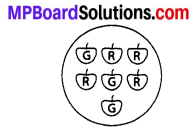Solution:
(a) Since total outcomes are A, A, B, C, D i.e., total 5 outcomes.
D occurs only once in a spinning wheel. Then, probability of the pointer stopping on D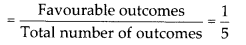(b) Number of aces = 4 Total number of cards = 52
∴ Probability of getting an ace from a well shuffled deck of 52 playing cards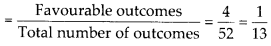(c) Number of red apples = 4
Total number of apples = 7
∴ Probability of getting a red apple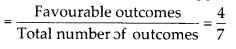Question 4.
Numbers 1 to 10 are written on ten separate slips (one number on one slip), kept in a box and mixed well. One slip is chosen from the box without looking into it. What is the probability of
(i) getting a number 6?
(ii) getting a number less than 6?
(iii) getting a number greater than 6?
(iv) getting a 1-digit number?
Solution:
Total number of outcomes = 10
(i) Favourable outcomes of getting a number 6 = 10
∴ Probability of getting a number 6
Favourable outcomes of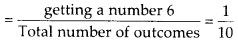(ii) Favourable outcomes of getting a number less than 6 = 5, i.e., 1, 2, 3, 4, 5
∴ Probability of getting a number less than 6
Favourable outcomes of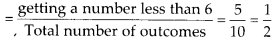(iii) Favourable outcomes of getting a number greater than 6 = 4, i.e., 7, 8, 9, 10
∴ Probability of getting a number greater than 6
Favourable outcomes of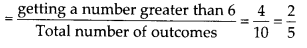(iv) Favourable outcomes of getting a 1-digit number = 9, i.e, 1, 2, 3, 4, 5, 6, 7, 8, 9 ∴ Probability of getting a 1-digit number
Favourable outcomes of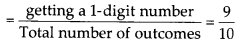Question 5.
If you have a spinning wheel with 3 green sectors, 1 blue sector and 1 red sector, what is the probability of getting a green sector? What is the probability of getting a non blue sector?
Solution:
Total number of outcomes = 5
Favourable number of outcomes of getting a green sector = 3
Favourable number of outcomes of getting a non-blue sector = 4
Thus, probability of getting a green sector
Favourable outcomes of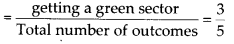Probability of getting a non-blue sector
Favourable outcomes of getting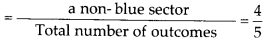Question 6.
Find the probabilities of the events given in Question 2.
Solution:
If Total number of outcomes = 6
(i) (a) Probability of getting a prime number
Favourable outcomes of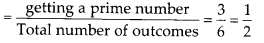(b) Probability of not getting a prime number
Favourable outcomes of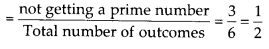(ii) (a) Probability of getting a number greater than 5
Favourable outcomes of getting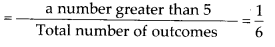(b) Probability of getting a number not greater than 5
Favourable outcomes of getting